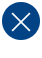Introduction to Rate Monotonic Scheduling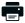Printable PDF

If you've got a lot of real-time tasks and tight deadlines, what's the best way to prioritize them? A technique called RMA guides the way.

The scheduling algorithm you choose depends on your goals. Different algorithms yield different results. Let's suppose you're given ten jobs and each will take a day to finish. In ten days, you will have all of them done. But what if one or more has a deadline? If the ninth task given to you has a deadline in three days, then doing the tasks in the order you receive them will cause you to miss that deadline.

The purpose of a real-time scheduling algorithm is to ensure that critical timing constraints, such as deadlines and response time, are met. When necessary, decisions are made that favor the most critical timing constraints, even at the cost of violating others. Real-time scheduling is also used to allocate processor time between tasks in soft real-time embedded systems.

Priority-based scheduling

Many real-time systems use preemptive multitasking, especially those with an underlying real-time operating system (RTOS). Relative priorities are assigned to tasks, and the RTOS always executes the ready task with highest priority.

In this case, the scheduling algorithm is the method in which priorities are assigned. Most algorithms are classified as static priority, dynamic priority, or mixed priority. A static-priority algorithm assigns all priorities at design time, and those priorities remain constant for the lifetime of the task. A dynamic-priority algorithm assigns priorities at runtime, based on execution parameters of tasks, such as upcoming deadlines. A mixed-priority algorithm has both static and dynamic components. Needless to say, static-priority algorithms tend to be simpler than algorithms that must compute priorities on the fly.

To demonstrate the importance of a scheduling algorithm, consider a system with only two tasks, which we'll call Task 1 and Task 2. Assume these are both periodic tasks with periods T1 and T2, and each has a deadline that is the beginning of its next cycle. Task 1 has T1 = 50 ms, and a worst-case execution time of C1 = 25 ms. Task 2 has T2 = 100 ms and C2 = 40 ms. Note that the utilization, Ui, of task i is Ci/Ti. Thus U1 = 50% and U2 = 40%. This means total requested utilization U = U1 + U2 = 90%. It seems logical that if utilization is less than 100%, there should be enough available CPU time to execute both tasks.

Let's consider a static priority scheduling algorithm. With two tasks, there are only two possibilities:

• Case 1: Priority(t1) > Priority(t2)
• Case 2: Priority(t1) < Priority(t2)

The two cases are shown in Figure 1. In Case 1, both tasks meet their respective deadlines. In Case 2, however, Task 1 misses a deadline, despite 10% idle time. This illustrates the importance of priority assignment.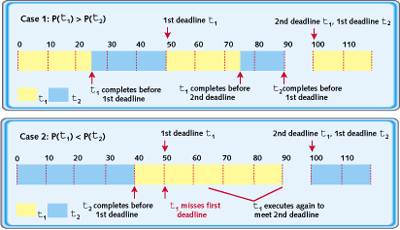Figure 1. Both possible outcomes for static-priority scheduling with two tasks (T1=50, C1=25, T2=100, C2=40)

Setting priorities

The rate monotonic algorithm (RMA) is a procedure for assigning fixed priorities to tasks to maximize their "schedulability." A task set is considered schedulable if all tasks meet all deadlines all the time. The algorithm is simple:

Assign the priority of each task according to its period, so that the shorter the period the higher the priority.

In the example, the period of Task 1 is shorter than the period of Task 2. Following RMA's rule, we assign the higher priority to Task 1. This corresponds to Case 1 in Figure 1, which is the priority assignment that succeeded in meeting all deadlines.

RMA is the optimal static-priority algorithm. If a task set cannot be scheduled using the RMA algorithm, it cannot be scheduled using any static-priority algorithm.

DVD Lecture: How to Prioritize RTOS Tasks (and Why it Matters)

One major limitation of fixed-priority scheduling is that it is not always possible to fully utilize the CPU. Even though RMA is the optimal fixed-priority scheme, it has a worst-case schedulable bound of:

Wn = n * (21/n - 1)

where n is the number of tasks in a system. As you would expect, the worst-case schedulable bound for one task is 100%. But, as the number of tasks increases, the schedulable bound decreases, eventually approaching its limit of about 69.3% (ln 2, to be precise).

It is theoretically possible for a set of tasks to require just 70% CPU utilization in sum and still not meet all their deadlines. For example, consider the case shown in Figure 2. The only change is that both the period and execution time of Task 2 have been lowered. Based on RMA, Task 1 is assigned higher priority. Despite only 90% utilization, Task 2 misses its first deadline. Reversing priorities would not have improved the situation.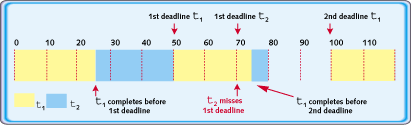Figure 2. Some task sets aren't schedulable (T1=50, C1=25, T2=75, C2=30)

In this case, the only way to meet all deadlines is to use a dynamic scheduling algorithm, which, because it increases system complexity, is not available in many commercial RTOSes.

Sometimes a particular set of tasks wil have total utilization above the worst-case schedulable bound and still be schedulable with fixed priorities. Figure 1's Case 1 is a perfect example. Schedulability then depends on the specifics of the periods and execution times of each task in the set, which must be analyzed by hand. Only if the total utilization is less than Wn can you skip that step and assume that all the tasks will meet all their deadlines.

RMA Guidelines

To benefit most from using a fixed-priority preemptive RTOS, consider the following rules of thumb:

• Always assign priorities according to RMA. Manually assigning fixed priorities will not give you a better solution.
• If total utilization is less than or equal to Wn, all tasks will meet all deadlines, so no additional work needs to be done.
• If total utilization is greater thanWn, an analysis of the specific task set is needed, to verify whether or not it will be schedulable.
• To achieve 100% utilization when using fixed priorities, assign periods so that all tasks are harmonic. This means that for each task, its period is an exact multiple of every other task that has a shorter period.

The last rule of thumb provides a simple guideline for most efficient use of the processor. For example, a three-task set whose periods are 10, 20, and 40, respectively, is harmonic, and preferred over a task set with periods 10, 20, and 50.

This article was published in the March 2002 issue of Embedded Systems Programming. If you wish to cite the article in your own work, you may find the following MLA-style information helpful:

Stewart, David and Michael Barr. "Rate Monotonic Scheduling," Embedded Systems Programming, March 2002, pp. 79-80.

Related Barr Group Courses:

Embedded Software Boot Camp

For a full list of Barr Group courses, go to our Course Catalog. See our Training Calendar for our latest public training calendar.

Rate monotonic scheduling

Thanks for the clear and concise explanation of RMA. In the scenario where two tasks have the same period but differing (average and worst-case) times of execution, what would be the correct way to assign priority?

Always Worst-Case

In RMA, only the worst-case matters. It's just as though the average case doesn't even exist!

First, you must consider the worst-case periods of the two tasks.  If both share a common worst-case in this respect, then they can either have equal priorities or adjacent priorities (in either order).

Only then, will you look at the execution time (again worst case).  These timings only matter for the calculation of CPU utilization, which has an upper limit.